Next: Plane Waves Up: Wave-Particle Duality Previous: Introduction

# Wavefunctions

A wave is defined as a disturbance in some physical system which is periodic in both space and time. In one dimension, a wave is generally represented in terms of a wavefunction: e.g.,(27)

whererepresents position,represents time, and,,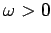. For instance, if we are considering a sound wave then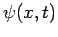might correspond to the pressure perturbation associated with the wave at positionand time. On the other hand, if we are considering a light wave thenmight represent the wave's transverse electric field. As is well-known, the cosine function,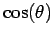, is periodic in its argument,, with period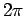: i.e.,for all. The function also oscillates between the minimum and maximum values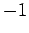and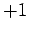, respectively, asvaries. It follows that the wavefunction (27) is periodic inwith period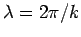: i.e.,for alland. Moreover, the wavefunction is periodic inwith period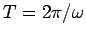: i.e.,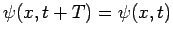for alland. Finally, the wavefunction oscillates between the minimum and maximum values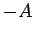and, respectively, asandvary. The spatial period of the wave,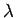, is known as its wavelength, and the temporal period,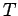, is called its period. Furthermore, the quantityis termed the wave amplitude, the quantitythe wavenumber, and the quantitythe wave angular frequency. Note that the units ofare radians per second. The conventional wave frequency, in cycles per second (otherwise known as hertz), is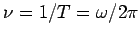. Finally, the quantity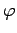, appearing in expression (27), is termed the phase angle, and determines the exact positions of the wave maxima and minima at a given time. In fact, the maxima are located at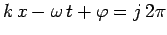, where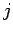is an integer. This follows because the maxima ofoccur at. Note that a given maximum satisfies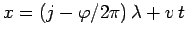, where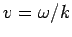. It follows that the maximum, and, by implication, the whole wave, propagates in the positive-direction at the velocity. Analogous reasoning reveals that(28)

is the wavefunction of a wave of amplitude, wavenumber, angular frequency, and phase angle, which propagates in the negative-direction at the velocity.Next: Plane Waves Up: Wave-Particle Duality Previous: Introduction
Richard Fitzpatrick 2010-07-20